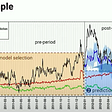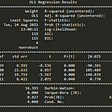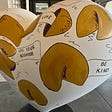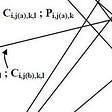# Statistics (II) in Data Science & Machine Learning

The first article on Statistics prepares us for the basics needed for data science. In the second article, we will study topics that build on these fundamentals. They will include the paired t-test, Sign test, Wilcoxon signed-rank test, two-sample t-test, degree of freedom, Chi-squared distribution, Chi-squared test, Fisher’s Exact Test, Risk, Bootstrap Hypothesis Testing, and Permutation Hypothesis Testing.

# Paired/Dependent Groups v.s. Independent Groups

A two-sample test collects one sample each from two groups. These two groups can be dependent or independent. In dependent groups, a member in one group is related to (paired with) a member in the other group. Indeed they can be the same person. For example, test scores are measured before and after a college preparation course. In a 2×2 crossover study, a subject receives two different treatments with a washout period in between. One-half of the subjects will start one treatment first while the other half start with the other. In matched groups, a subject in one group is paired with another subject. For factors that could impact the outcome, these two subjects will be exposed to (or possess) the same factors except the one that is under study.

In independent groups, sample points between samples are unrelated. They may be collected from two different populations that have no overlap and are different from the other in one key aspect. For example, the population is exposed or not exposed to a condition or a risk factor. Or subjects are randomly assigned to two different groups, one with treatment and one without.

# Paired t-test

Can we establish experiments to study the effect of a single factor while keeping other variables the same? How can we compare apples with apples?

Paired groups allow data points to be compared directly. In the example below, we subtract the “score before the SAT preparation course” from “score after the SAT preparation course”. Then we perform the paired t-test to check whether the preparation course is effective. Is the score difference (d) statistically significant?

The null hypothesis will be the difference in scores is zero.

The test statistic is the t-score on the sample mean on d:

With the t-score, we look up the p-value from a one-tailed table and draw a conclusion on statistical significance. Alternatively, we can compute the confidence interval on d.

# Sign Test

Paired t-test is a parametric test. In the last example, we model the sample mean of d with a t-distribution. To establish the model, we collect a reasonably large sample to estimate the standard error. If d is not normally distributed, we should worry about the accuracy for a sample size smaller than 20.

On the other hand, a non-parametric test, like the Sign test and Wilcoxon signed-rank test, requires a smaller sample size. It has no assumption on the data distribution for d. Findings are based on the sample data alone. But as shown later, some information is lost (truncated) which leads to lower power.

In the null hypothesis, we assume the sample median for d equals 0. i.e. half of the paired differences are greater or equal to zero and the other half smaller or equal to zero.

For hypothesis testing, we can perform a Sign test on the median. We will reject the null hypothesis if the number of people having better scores is not statistically significant.

The probability of having k out of N people with better scores is

Let’s say 8 out of ten people has improved score. For a two-sided test, the p-value accounts for as extreme or more extreme than 8 positive scores, and the results of 0, 1, or 2 positive scores.

# Wilcoxon Signed-rank test

However, how can we include the magnitude of the difference into our consideration? The magnitude of the difference may impact us in determining whether the difference is significant. This is what the Wilcoxon signed-rank test does.

After computing the d column below, we take its absolute value and rank it. For example, subject #8 below is ranked as second since its absolute difference is the second-lowest.

If the null hypothesis is true, we can add and compare the ranking for those with improved scores and those with deteriorated scores. The difference should not be statistically significant.

Specifically, we add the rank numbers with positive d and those with negative d respectively. We label these sums as sum+ and sum-. Without the sign, all the rank numbers add up to 55. If H₀ is true, half of the subjects have positive d and the other half have negative d. Therefore, the expected sum+ should equal the expected sum-. i.e. half of 55 (27.5). The probability of the observed result under the H₀ assumption is

p is normally distributed regardless of the problem we solve. We don’t need to build and estimate a distribution model for d. Without proof here, it has μ and σ² equal E[T⁺] and var(T⁺) below, which depend on our sample size n (n=10).

We use software to calculate this. But we can also solve it with the Wilcoxon Signed-Ranks table. With α and n, it provides the critical value for sum+ (or sum-) to reject H₀. This table is based on a normal distribution with the mean and variance above.

# Degrees of freedom (df)

To find the p-value from a t-distribution (t-score table), we need to know the degrees of freedom first. Actually, many distributions require df too.

The equation “y = mx + b” represents lines on the left below. By varying m and b, we get two degrees of freedom (df). This creates all possible lines in a 2-D plane.

By adding constraints, we reduce the df. For example, fixing b to 2, we reduce df by one. The lines represented have one single degree of freedom (m) now. All lines intersect at y=2. By further setting m = 0.5, df reduces to 0. We don’t have any choice but a straight line on the right above.

When we have a sample size of n, we get n random variables. We have n degrees of freedom. However, in some studies, we don’t know the population variance and need to estimate it from the sample. This reduces df by one.

If we don’t know the population variance, many tests use the sample variance S² instead. To compute S², we subtract the sample mean from each data point Xᵢ first.

The sample mean adds a constraint to the system. Without the loss of generality, let’s say the sample mean is 0 to make the equation simpler.

We can vary any n-1 variables (Xᵢ). But once they are chosen, the last remaining variable is fixed to fulfill the sample sum to be zero.

In general, to model a distribution, if it requires k distinct parameters (statistics) derived from the sample data, df equals n-k. In t-distribution (T), we use the sample mean to calculate S. Therefore, the degrees of freedom drop by 1. df equals n-1.

# Two-sample t-test

The variances of two different groups can be assumed to be the same or different. In the examples discussed before, we assume they are the same. This is an assumption that researchers make before a study. But researchers can assume they are different. In this section, we will discuss how to estimate the standard error under both assumptions.

Unequal variances t-test (Welch’s t-test)

Let’s assume the researchers believe the variances for the two groups in the study are different.

Consider the data below

And the testing hypotheses are

which H₀ assumes the sample means for both groups are the same.

Let’s consider a random variable U derived from their difference

The variance for U is

The standard error SE equals the square root of this variance. It is the SE for the sampling distribution for the difference in the sample means between Group a and b.

And we can use it to calculate the t-score on the sampling difference.

In our example, the S.E. normalizes a difference of 20 when it is expected to be zero. Given this score, we can find the p-value and determine whether we will reject H₀.

The degree of freedom (df) equals

and we can find the p-value from a t-distribution with the df computed above.

Equal variance t-test

Researchers may assume both groups should have equal variance if there is no reason to believe otherwise. Any difference in the calculated variances between groups is caused by sampling variability.

To compute SE, we first compute a pooled variance. This is a weighted sum of the variances calculated in each group.

The standard error for the sampling distribution will be:

Using the previous example, the test statistic t-score on the sample mean is

As another side note, df equals

# Chi-squared distribution (χ2 distribution)

Before moving to more complex statistical methods, let’s model another distribution first. The key idea is if certain random variables follow one of these distributions, we can use the corresponding pdf to compute the p-value.

If Z₁, Z₂, …, and Zk are k independent random variables that each with a standard normal distribution. The random variable Q holding its sum

will have a distribution called chi-squared distribution 𝓧² with k degrees of freedom.

Here is the pdf for the chi-squared distribution.

From a chi-squared table, we can look up the critical chi-squared score for a given degrees of freedom and α. If a computed score is greater than this critical value, we will reject H₀.

# Chi-squared test

Chi-squared tests determine whether two variables are related or not. For example, it determines whether gender influences the choice to have a dog or a cat as a pet. The hypotheses are

The contingency table below counts the number of cats and dogs to be adopted between males and females. This is the contingency table with the observed values.

First, we construct the counts in each cell if H₀ is true.

There is a shortcut in computing these expected cell values. Rᵢ is the total count in row i, Cⱼis the total count in column j and T is the grand total. For each cell, we multiple Rᵢ with Cⱼand divide it by T.

The counts in the parentheses below are the expected count in each cell if H₀ is true.

Let’s convert these values into a chi-squared score. If H₀ is correct, X² below is a chi-squared distribution as n approaches infinity.

The chi-squared statistic is therefore defined as

For the cell₁₁, it equals 51.43.

The chi-squared statistic is 160.71 in our example. We can consult the chi-squared table to find the p-value. Since the values of the cells in a row must add up to the total of males or females, it reduces the df by 1. The total in cats and dogs also reduces the df in the column. Therefore, df equals (# of rows-1) × (# of column-1) (=1×1). The p-value here is <0.00001. Therefore, we will reject the null hypothesis.

# Fisher’s Exact Test

The chi-squared test applies an approximation assuming the sample size is large. Fisher’s exact test is an exact test but with a much higher computation cost. When many counts in the contingency table are in the single-digit, Fisher’s exact test will be recommended as it will be more accurate.

Consider a jar with N balls. K balls are in red and N-K will be blue. If we are going to sample r balls without replacement, the chance of getting x red balls is

The denomination counts the combinations of sampling r balls out of N balls. The numerator is the combination of selecting x balls from the red times the combination of selecting r-x balls from the blue. So the total number of balls sampled is r.

In Fisher’s exact test, we can simply replace the “sampled” and the “unsampled” labels with the variable in interest (say, male and female).

Given N = 21, K = 9 and r = 12, p(2) equals

For a lower tail test, given x = 2, we sum up the case with 2 and less for the p-value. i.e the p-value will be p(2) + p(1) +p(0).

# Risk

In this section, we will introduce metrics in understanding risk factors.

The following table records the counts on how many people get and do not get a disease (D) if they have and have not been exposed (E) to a risk factor.

Then,

Here are some of the terms and calculations. The risk difference (RD) is

The exposure increases the risk by 10% (on the additive scale).

The relative risk (RR) is

It is 2 times as likely to get the disease after exposure or the exposure increases the risk by 100% on the multiplicative scale.

The odds ratio (OR) is

The odds of getting the disease for people with exposure is 2.25 times the odds for those without exposure.

For a case-control design, we study the exposure factor from those with or without the disease. Hence, the prevalence P(D|E) is unknown. Instead, we can trace people with disease or not disease and calculate P(E|D). Without proof here, the odds ratio can be computed from this perspective as they both have the same result mathematically.

# Bootstrapping

The variance of the sampling mean can be derived from the variance σ² of the data analytically.

But it may not obvious or possible for other test statistics like the median or the top 75% percentile. In other cases, the sample size is small and we need an alternative approach to understand the sampling distribution.

One possibility is to repeat the study over and over. In each trial, we find the statistic of interest. Eventually, we can collect enough data points to reconstruct the sampling distribution. We can also use these results to compute the SD of the sampling distribution. This approach has no assumption on whether the sampling distribution is normally distributed or not. It does not need any special equation. But repeating the experiments many times is usually expansive.

In bootstrapping, we only collect a sample once (say Sample C). Consequently, we resample from this sample with replacement. So instead of m independent samples, bootstrapping gets m resampling samples from C. Bootstrapping retains the simplicity in reconstructing the distribution without paying the cost of collecting new samples. CPU time is relatively cheap. We can perform the resampling over 10K times. We will not gain more information than what Sample C contains. But by repeating the resampling many times, we can construct a finer model on what Sample C suggests.

# Bootstrap Hypothesis Testing

In the example below, we collect data from two independent groups. For example, Group A exercises 60 minutes a day, and Group B exercises 30 minutes a day. We will collect SBP data for hypothesis testing with bootstrap.

In each bootstrap, we perform sampling with replacement. Note, we don’t consider the group membership in the resampling. Any data point can be selected from any group. As shown below, subject #1 belonging to Group A is now subject #9 belonging to Group B in Bootstrap 1. If H₀ is correct, we can swap members between groups without impacting the overall statistics. Group A and B are not different under H₀.

After creating b bootstrap, we compute the absolute difference between Group A and B for all the bootstraps. We compare them with that of the observed SBP. p-value equals

Intuitively, if the number of bootstraps that have a greater difference than the observed SBP is small, we should reject H₀. If H₀ is not true, bootstraps with a larger difference than the observed should appear with less frequency. The numerator above is small.

To find the confidence level for the estimation:

We do sampling with replacement again. But, we only sample from the same group now. By collecting many bootstraps, say b>10K, we can rebuild the sampling distribution and compute the standard error.

# Permutation Hypothesis Testing

Permutation Hypothesis testing is similar to bootstrap hypothesis testing. Instead of sampling with replacement, we shuffle the value regardless of the group membership.

The p-value is

# Next

Our final article on statistics is mainly on the topic of ANOVA. It allows us to handle multiple groups and multiple factors?

--

--

--

## More from Jonathan Hui

Deep Learning

Love podcasts or audiobooks? Learn on the go with our new app.

## Sourcing book data and domino effect## The Resume of : Inferring the Effect of an Event using CausalImpact by Kay Brodersen## ML-Running diagnostics## Linear Regression Fish Data Set## When Data is Scarce… Ways to Extract Valuable Insights## Data Science in Insurance## Credit Agricole Leverages the Denodo Platform to Streamline Data Access## Advanced Options with Hyperopt for Tuning Hyperparameters in Neural NetworksDeep Learning

## Statistics (III) ANOVA in Data Science & Machine Learning## The Practical Usefulness of Sparse Data Representation in Machine Learning## Optimizers in Machine Learning## Explainable AI (XAI): The Easy Guide for Beginners